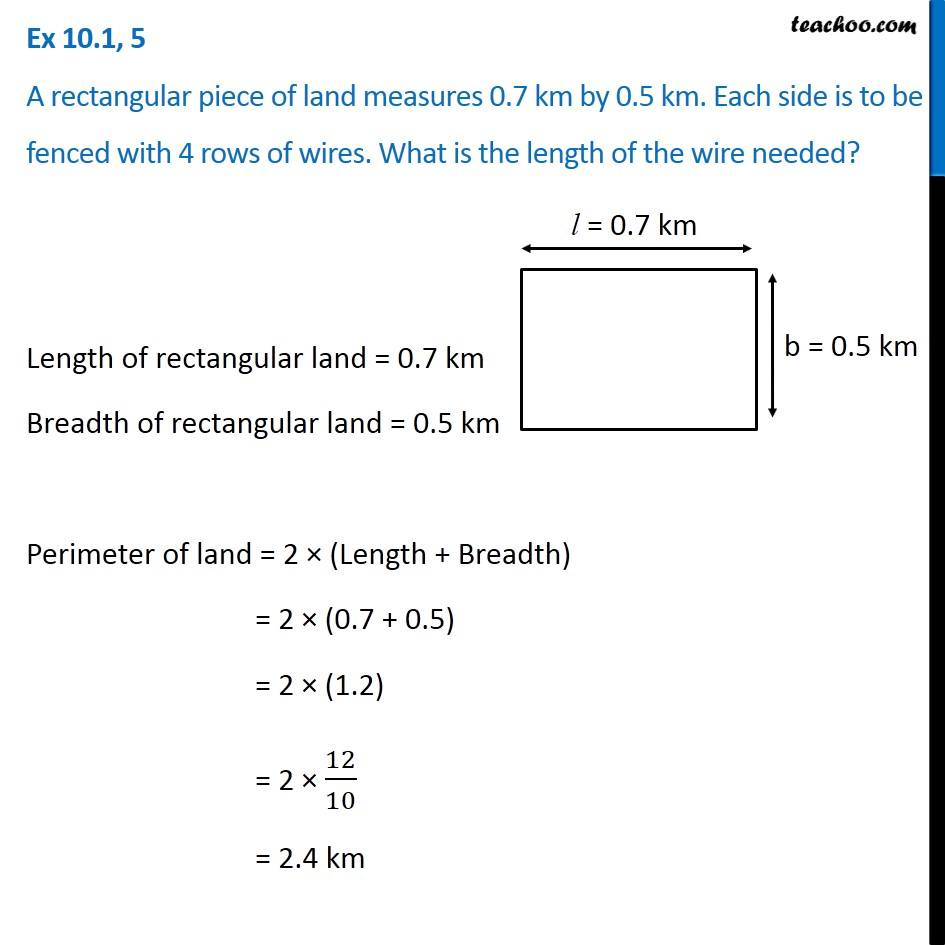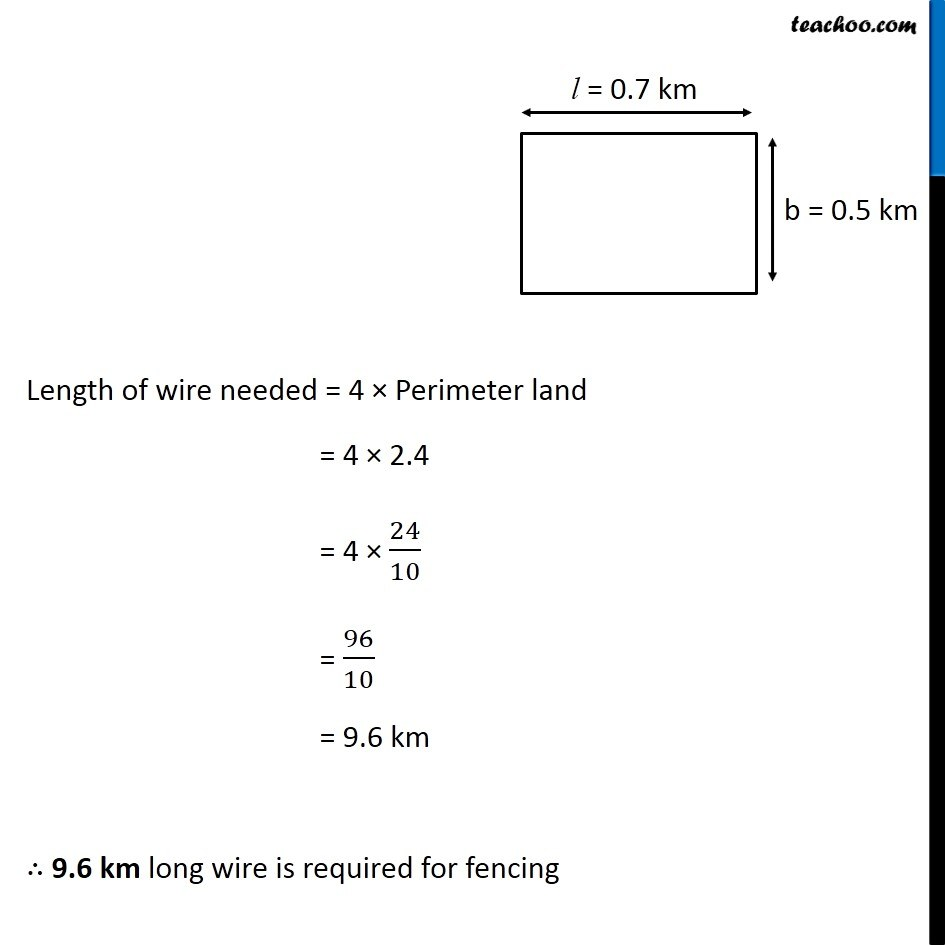Ex 10.1

Chapter 10 Class 6 Mensuration
Serial order wiseLearn in your speed, with individual attention - Teachoo Maths 1-on-1 Class

### Transcript

Ex 10.1, 5 A rectangular piece of land measures 0.7 km by 0.5 km. Each side is to be fenced with 4 rows of wires. What is the length of the wire needed? Length of rectangular land = 0.7 km Breadth of rectangular land = 0.5 km Perimeter of land = 2 × (Length + Breadth) = 2 × (0.7 + 0.5) = 2 × (1.2) = 2 × 12/10 = 2.4 km Length of wire needed = 4 × Perimeter land = 4 × 2.4 = 4 × 24/10 = 96/10 = 9.6 km ∴ 9.6 km long wire is required for fencing